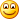# A bit on making functions tail-recursive in F#

If you recall a while back when I was demonstrating some Functional Data Structures, I mentioned the fact that some of the functions were not tail recursive, and that this is something that we would probably want to do something about. Which raises the question: How exactly do we go about making a function tail-recursive? I am going to attempt to address that question here.

One of the first problems with creating a tail recursive function is figuring out whether a function is tail recursive in the first place. Sadly this isn’t something that is always obvious. There has been some discussion about generating a compiler warning if a function is not tail recursive, which sounds like a dandy idea since the compiler knows enough to know how to optimize tail recursive functions for us. But we don’t have that yet, so we’re going to have to try and figure it out on our own. So here are some things to look for:

1. When is the recursive call made? And more importantly, is there anything that happens after it? Even something simple like adding a number to the result of the function can cause a function to not be tail-recursive
2. Are there multiple recursive calls? This sort of thing happens when processing tree-like data structures quite a bit. If you need to apply a function recursively to 2 sub-sets of elements and then combine them, chances are they are not tail-recursive
3. Is there any exception handling in the body of the function? This includes use and using declarations. Since there are multiple possible return paths then the compiler can’t optimize things to make the call recursive.

Now that we have a chance of identifying non-tail-recursive functions lets take a look at how to make a function tail-recursive. There may be cases where its not possible for various reasons to make a function tail-recursive, but it is worthwhile trying to make sure recursive functions are tail-recursive because a StackOverflowException cannot be caught, and will cause the process to exit, regardless (yes, I know this from previous experience)

### Accumulators

One of the primary ways of making a function tail-recursive is to provide some kind of accumulator as one of the function parameters so that you an build the final result and pass it on using the accumulator, so when the recursion is complete you return the accumulator. A very simple example of this would be creating a sum function on a list of ints. A simple non-tail-recursive version of this function might look like:

```let rec sum (items:int list) =
match items with
| [] –> 0
| i::rest –> i + (sum rest)
```

And making it tail-recursive by using an accumulator would look like this:

```let rec sum (items:int list) acc =
match items with
| [] –> acc
| i::rest –> sum rest (i+acc)
```

### Continuations

If you can’t use an accumulator as part of your function another (reasonably) simple approach is to use a continuation function. Basically the approach here is to take the work you would be doing after the recursive call and put it into a function that gets passed along and executed when the recursive call is complete. For an example where we’re going to use the insert function from my Functional Data Structures post. Here is the original function:

```let rec insert value tree =
match (value,tree) with
| (_,Empty) –> Tree(Empty,value,Empty)
| (v,Tree(a,y,b)) as s –>
if v < y then
Tree(insert v a,y,b)
elif v > y then
Tree(a,y,insert v b)
else snd s
```

This is slightly more tricky since we need to build a new tree with the result, and the position of the result will also vary. So lets add a continuation function to this and see what changes:

```let rec insert value tree cont =
match (value,tree) with
| (_,Empty) –> Tree(Empty,value,Empty) |> cont
| (v,Tree(a,y,b)) as s –>
if v < y then
insert v a (fun t –> Tree(t,y,b)) |> cont
elif v > y then
insert v b (fun t –> Tree(a,y,t)) |> cont
else snd s |> cont
```

For the initial call of this function you’ll want to pass in the built-in id function, which just returns whatever you pass to it. As you can see the function is a little more involved, but still reasonably easy to follow. The key is to make sure you apply the continuation function to the result of the function call, otherwise things will fall apart pretty quickly

These two techniques are the primary means of converting a non-tail-recursive function to a tail-recursive function. There is also a more generalized technique known as a “trampoline” which can also be used to eliminate the accumulation of stack frames (among other things). I’ll leave that as a topic for another day, though.

Another thing worth pointing out, is that the built-in fold functions available in the F# standard library are already tail-recursive. So if you make use of fold you don’t have to worry about how to make your function tail recursive. Yet another reason to make fold your go-to function.

• Jay Robinson

Hey, you’re a pain to try to reach! I hope this actually gets to you. Find me somehow, downshiftdata@anywhere. J